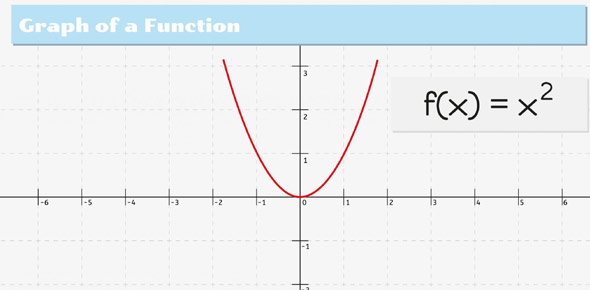# Linear Functions Pre-test Questions

Approved & Edited by ProProfs Editorial Team
At ProProfs Quizzes, our dedicated in-house team of experts takes pride in their work. With a sharp eye for detail, they meticulously review each quiz. This ensures that every quiz, taken by over 100 million users, meets our standards of accuracy, clarity, and engagement.
| Written by Symphony1234
S
Symphony1234
Community Contributor
Quizzes Created: 1 | Total Attempts: 644
Questions: 5 | Attempts: 644SettingsThis is a basic assessment on linear functions. It is designed as a basic tool to find the skill level of students in this area.

• 1.

### What is the formula for slope?

• A.

Rise over run

• B.

Run over rise

• C.

X over Y

• D.

Y-intercept times X-intercept divided by pie

• E.

The opposite of the X-intercept

A. Rise over run
Explanation
The formula for slope is rise over run. This means that to calculate the slope of a line, you divide the vertical change (rise) by the horizontal change (run) between any two points on the line. The rise over run formula allows us to quantify the steepness or incline of a line and is a fundamental concept in mathematics and physics.

Rate this question:

• 2.

### What formula describes the line presented to you on the board? (in slope intercept form)

• A.

Y=2x+6

• B.

Y=x+3

• C.

2x+3y=17

B. Y=x+3
Explanation
The correct answer is y=x+3. This is because the equation is already in slope-intercept form, which is y=mx+b, where m represents the slope and b represents the y-intercept. In this case, the slope is 1 (since the coefficient of x is 1) and the y-intercept is 3. Therefore, the equation y=x+3 describes the line presented on the board.

Rate this question:

• 3.

### What is '2x+3y=18' in slope intercept form?

• A.

Y=-2x+18

• B.

Y=-(2/3)x+18

• C.

Y=-(2/3)x+6

• D.

Y=2x+6

• E.

Y=(2/3)x+18

C. Y=-(2/3)x+6
Explanation
The equation '2x+3y=18' can be rearranged into slope-intercept form, which is y=mx+b, where m represents the slope and b represents the y-intercept. By isolating the y-term, we can rewrite the equation as y=-(2/3)x+6. This means that the slope of the line is -2/3 and the y-intercept is 6.

Rate this question:

• 4.

### The form of a function that is written as ax+by=c is __________ form.

standard
Explanation
The form of a function that is written as ax+by=c is called the standard form. In this form, the coefficients of x and y are represented by a and b respectively, and c is the constant term. Standard form is often used in mathematics to represent linear equations in two variables.

Rate this question:

• 5.

### Answer the question that you are given.

Related TopicsBack to top
×

Wait!
Here's an interesting quiz for you.